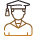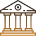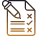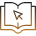# Derivatives And Economics: How They Help Analyse Market Trends?

###### ByRamraj Saini###### Synopsis

Economics is like a big puzzle where we figure out how to use limited resources to make everyone's lives better. Derivatives are like special tools in this economic puzzle. This article explores how these tools, and derivatives, shape the world of economics and help us understand what's happening in today's markets. To gain deeper insight on this, keep reading.###### Synopsis

Economics is like a big puzzle where we figure out how to use limited resources to make everyone's lives better. Derivatives are like special tools in this economic puzzle. This article explores how these tools, and derivatives, shape the world of economics and help us understand what's happening in today's markets. To gain deeper insight on this, keep reading.

Economics is like a big puzzle where we figure out how to use limited resources to make everyone's lives better. It's about how people, businesses, and even whole countries make choices about what to produce, buy, and sell.

Derivatives are like special tools in this economic puzzle. It is a financial instrument that helps us deal with money and risks. Think of it as tools that can predict the future prices of things like stocks, bonds, and other important stuff.

In our journey, we'll see how derivatives and economics work together to help us understand and shape the world of money and markets.

## Maths Behind Derivatives

Derivatives may sound complicated, but it is essentially about understanding how things change. This is where maths, especially calculus, comes into play.

>> Calculus is a branch of maths that deals with change. Imagine you're on a roller coaster; calculus helps us understand how fast you're going up or down at any given moment. Similarly, derivatives tell us how fast something is changing.

>> Derivative of a Function In simple terms, the derivative of a function tells us how that function changes as we tweak its inputs. If you have a function like f(x), its derivative, written as f'(x), measures how fast f(x) is changing as x changes.

Let's say we have a function f(x) = 3x2 + 2x - 1. Find the derivative f'(x).

To find f'(x), we'll apply the power rule of differentiation. For each term in the function, we'll multiply the coefficient by the exponent and then reduce the exponent by 1.

Derivative of 3x2: 2 * 3x(2-1) = 6x

Derivative of 2x: 1 * 2x(1-1) = 2

Derivative of -1: 0 (since it's a constant)

So, f'(x) = 6x + 2.

To calculate derivatives, we use formulas like the power rule, sum and difference rule, product rule, and quotient rule. These formulas are like magic spells for solving derivative problems.

Sum Rule:

d/dx (f(x) + g(x))] = [d/dx (f(x))] + [d/dx (g(x))]

Difference Rule:

[d/dx (f(x) - g(x))] = [d/dx (f(x))] - [d/dx (g(x))]

Product Rule: [d/dx (f(x) * g(x))] = [d/dx f(x)] * g(x) + f(x) * [d/dx g(x)]

Quotient Rule: [d/dx (f(x)/g(x))] = ([d/dx f(x)] * g(x) - f(x) * [d/dx g(x)]) / [g(x)]2

## Standard Derivatives

[d/dx (xn)] = n * x(n-1)

[d/dx (sin(x))] = cos(x)

[d/dx (cos(x))] = -sin(x)

[d/dx (tan(x))] = sec2(x)

[d/dx (cot(x))] = -csc2(x)

[d/dx (sec(x))] = sec(x) * tan(x)

[d/dx (csc(x))] = -csc(x) * cot(x)

[d/dx (ax)] = ax * ln(a)

[d/dx (ex)] = ex

[d/dx (ln(x))] = 1/x

For students to grasp the concepts of derivatives and their significance in economics, this article serves as an invaluable resource. Whether you're a Class 11 or Class 12 student curious about where these topics fit into your curriculum or someone preparing for competitive exams, you'll find clarity here.

These essential concepts are often covered in the mathematics and economics chapters such as Class 11, in Chapter 13 “Limit and Derivatives” and understanding them can be a game-changer in your academic journey. It simplifies complex ideas, offers practical examples, and ignites curiosity, ultimately helping students gain a deeper understanding and excel in your studies.

## Derivatives For Risk Management

Derivatives aren't just about maths; they're superheroes when it comes to managing risk. Here's how maths helps us use derivatives for this purpose:

### Hedging with Derivatives

>>Protecting a Farmer's Crops: Imagine a farmer worried about the prices of their crops falling before harvest. With derivatives, they can create a mathematical shield. By signing a futures contract to sell their crops at a set price, they're like wizards ensuring they won't lose money, no matter what the market does.

### Currency Hedging

>> A Company Dealing in Different Currencies: Global businesses often deal with different currencies, and exchange rates can be tricky. But don't worry, maths helps them too! With derivatives like forward contracts, they use mathematical strategies to secure favourable exchange rates for future transactions.

### Interest Rate Derivatives

>> Banks and Interest Rates: Banks use derivatives to tackle interest rate changes. Maths helps them balance their books by aligning interest rates on loans and deposits, keeping everything in financial harmony.

## Maths Unveils Market Trends

Derivatives are like magic glasses that help us see market trends and economic mysteries. Here's how maths makes it happen:

### Mathematical Clues to Market Sentiment

Derivatives markets are full of mathematical indicators. These are like treasure maps, showing us where the market might be heading.

>> Predicting Stock Movements: For stocks, we have indicators like the Black-Scholes model. It uses maths to tell us if a stock's price might go up or down based on factors like implied volatility. It's like having a crystal ball for stocks.

An economist is studying the relationship between a company's advertising spending (x) and its quarterly sales (y). After analysing the data, the economist develops the following mathematical model for the relationship: y = 2x2 + 3x + 10. Find the rate of change in sales with respect to advertising spending when the company spends ₹50,000 on advertising.

We need to find the derivative dy/dx of the sales (y) with respect to advertising spending (x).

Using the power rule for each term

dy/dx = 4x + 3.

Now, we can plug in x = 5,000 (the advertising spending) into our derivative:

dy/dx at x = 50,000

= 4 * 50,000 + 3

= 2,00,000 + 3

= 2,00,003.

So, when the company spends ₹50,000 on advertising, the rate of change of sales with respect to advertising spending is ₹2,00,003 per unit of advertising spending.

### Maths and Market Volatility

>> Maths Behind Volatility: Volatility measures how much prices swing around. Derivatives tied to volatility, such as the VIX (Volatility Index), rely on complex maths models. They predict when the market might get stormy, helping traders prepare.

### Studying the 2008 Financial Crisis

Credit default swaps are like insurance policies for loans and bonds. They were used by banks and financial institutions to protect themselves against the risk of borrowers not being able to repay their debts. However, when the housing market collapsed, and many homeowners couldn't make their mortgage payments, these CDS contracts came into play.

The crisis erupted because the scale of these CDS contracts was enormous, far exceeding the actual value of the underlying loans and bonds. It was like insuring a house for a million dollars when it was only worth a hundred thousand. When the housing market crumbled, the banks faced massive losses that they couldn't cover.

This is where maths and derivatives played a crucial role. Economists and financial experts used complex mathematical models to dissect the web of interconnected CDS contracts and understand the potential fallout. These models helped in unravelling the puzzle of how the crisis had spread so rapidly and extensively throughout the financial system.

The insights gained from these mathematical analyses were instrumental in devising new rules and regulations to make financial markets safer and prevent such a catastrophic event from happening again.

## Recent Market Trends

Mathematics is at the heart of understanding recent market trends using derivatives. Let's explore some trends.

### Cryptocurrency Magic

Cryptocurrencies, with Bitcoin leading the way, have revolutionised the financial world. These digital assets have captured the imagination of investors and technophiles alike. However, beneath the surface of this digital gold rush lies a world driven by mathematics.

Mathematics is the language of cryptocurrencies, helping us calculate essential aspects such as the fair value of Bitcoin futures. These calculations consider factors like supply and demand, historical price movements, and market sentiment. In essence, maths allows us to make informed decisions when buying, selling, or holding cryptocurrencies.

Moreover, understanding the risks and rewards of investing in digital currencies requires a keen mathematical eye. It involves assessing market volatility, analysing price charts, and calculating potential gains or losses. In this brave new world, maths is not just a tool but a necessity for those seeking to navigate the cryptocurrency landscape.

### Low-Interest Rates

The global financial landscape has witnessed a historic era of low-interest rates. Central banks worldwide have adopted strategies to keep interest rates at record lows in response to economic challenges. In this environment, mathematics becomes the guiding star for financial institutions.

Mathematics helps financial institutions design and implement strategies to maximise their returns in a low-yield environment. For example, they use mathematical models to determine the optimal mix of assets in their portfolios. These models consider various factors, including interest rates, inflation, and risk tolerance. By harnessing the power of maths, financial institutions can adapt and thrive despite the challenging interest rate landscape.

### Sustainable Finance

Sustainability has emerged as a paramount concern in recent years, with businesses and investors increasingly focusing on environmental and social responsibilities. Within this framework, mathematics plays a crucial role in instruments like carbon emission credits.

Carbon credits are a mathematical solution to combat climate change. These credits are allocated based on a formula that considers a company's emissions and its efforts to reduce them. Investors and businesses use mathematical models to assess the value and impact of these credits. Maths empowers them to make environmentally responsible decisions, encouraging eco-friendly practices and investments.

## Careers360 helping shape your Career for a better tomorrow#### 250M+

Students#### 30,000+

Colleges#### 500+

ExamsE-Books